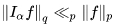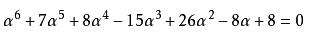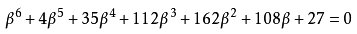1.概念陈述

2.例子

lim(x->0)（x^2*e^(1/x^2)）
=lim(x->0) e^(1/x^2)/(1/x^2)
=lim(t->∞) e^t/t
=lim(t->∞) e^t
＝∞

S(ψ,q)=∑eq(ψ(x))，x∈(1,q)，x∈N+.

S(R,q)=∑eq(R(x))，x∈(1,q)，x∈N+.α=(−7+9y−33w−24w+3yw−2yw)/23，β=(−27−8y−9w+6w−18yw−11yw)/23.

α和β也是代数整数，满足：3.小结

posted on 2018-03-24 15:56  milantgh  阅读(164)  评论(0编辑  收藏